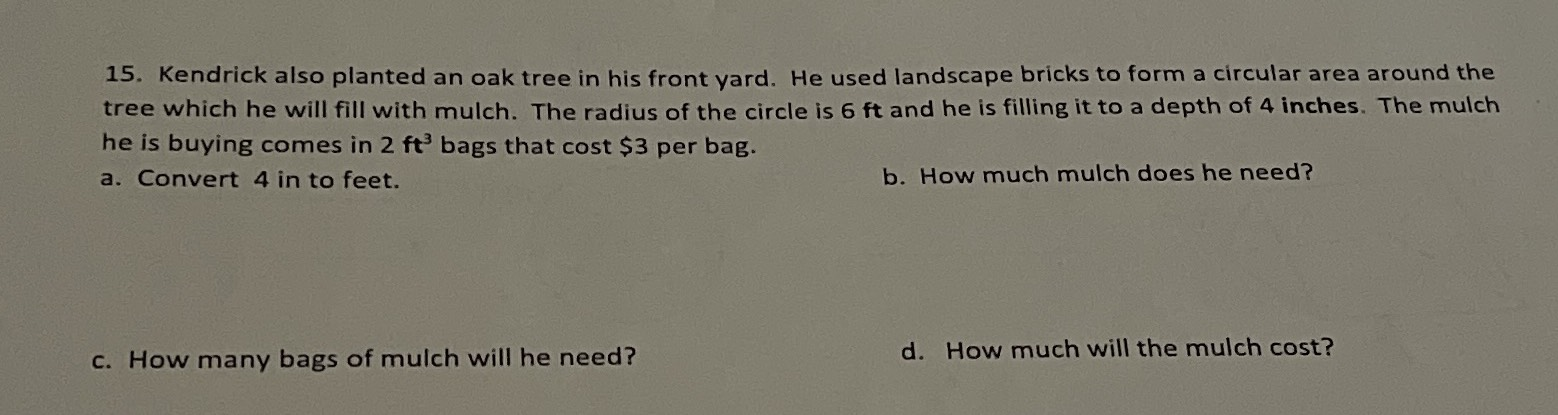### ¿Todavía tienes preguntas de matemáticas?

Pregunte a nuestros tutores expertos
Algebra
Pregunta15. Kendrick also planted an oak tree in his front yard. He used landscape bricks to form a circular area around the tree which he will fill with mulch. The radius of the circle is $$6$$ ft and he is filling it to a depth of $$4$$ inches. The mulch he is buying comes in $$2$$ ft $$3$$ bags that cost $$\ 3$$ per bag.

a. Convert $$4$$ in to feet.

b. How much mulch does he need?

c. How many bags of mulch will he need?

d. How much will the mulch cost?